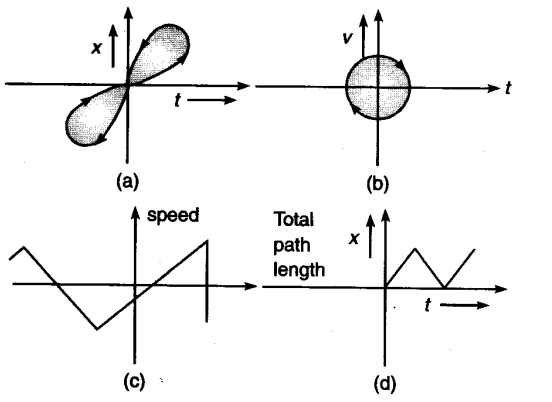# State with reasons which of the following cannot possibly represent one dimensional motion of a particle

State with reasons which of the following cannot possibly represent one dimensional motion of a particle.. Figure (a) does not represent one dimensional motion of particle because the particle has two different positions at the same instant which is not the case of one dimensional motion. Figure (d) also does not represent one dimensional motion of the particle because here the total path length is shown to decrease with time which is not possible in one dimensional motion.
Graph (b) does not represent one dimensional motion because at the same instant a particle cannot have positive and negative velocity if the motion is one dimensional.
Graph © does not represent one dimensional motion because speed cannot be negative as shown in graph

1 Like

Is above 4 situation is possible in 2-D